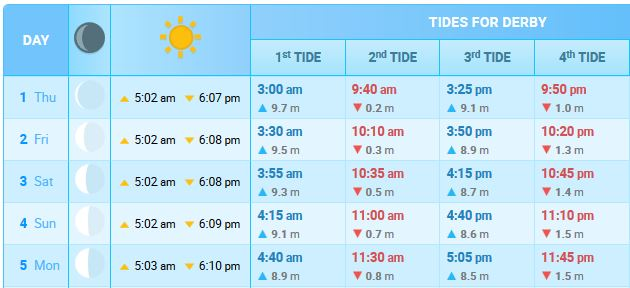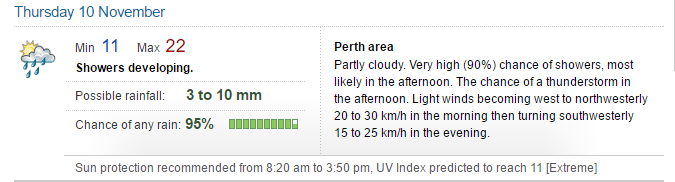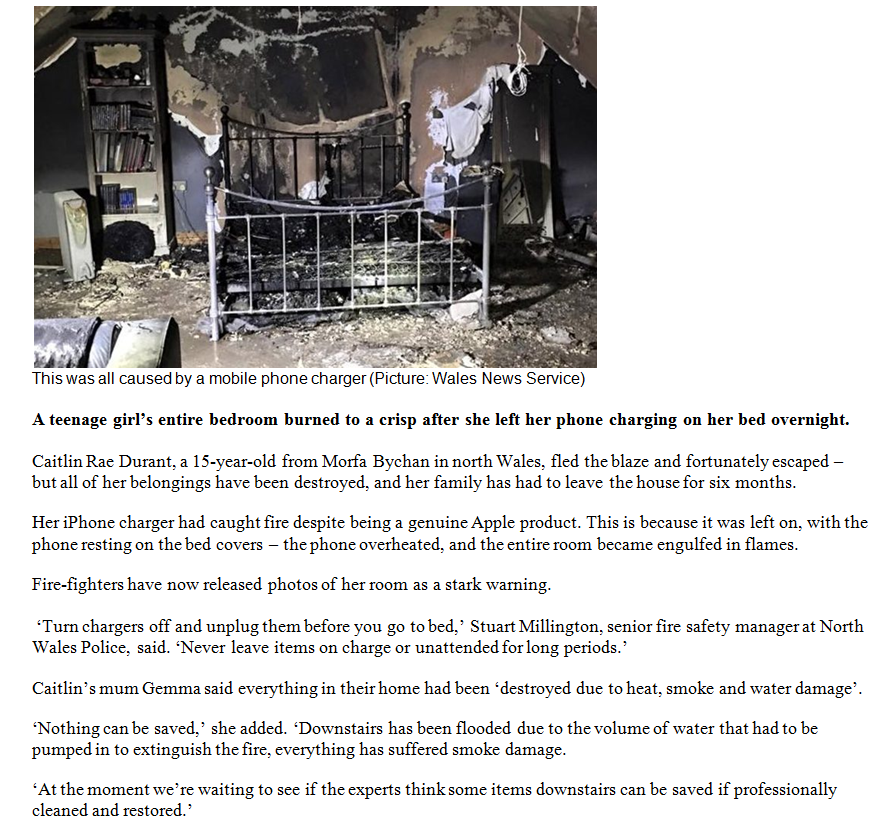Select Page
N

N

Step 2

Choose the answer you think is correct. Stuck? Click on “Explanation of Answer” for a step-by-step guide to answer the question or the “hint” if you need a clue
N

Step 3

Click Check Answer to instantly see if you are correct, otherwise click next. Please note you cannot change your answer once you’ve checked!

Welcome to the OLNA Support Example Skill Builder

• Each question will be multiple choice
• There will be 4 answers to select from
• If you can’t figure out the answer, you should use the “Explanation of the Answer” as a guide to work out the correct response

Click next to begin!

Question 72.

The table below shows the height, in metres, of the tides at Derby for five days in December 2016.

Low tides are printed in red and high tides are printed in blue.What was the difference between the highest and the lowest tides on Saturday, 3rd of December?

Step 1: In the table look for the row Saturday 3rd of December. Scan across and look for the red and blue digits. The tide height is under the coloured digits. The arrow pointing up is the high tide and the arrow pointing down is the low tide.

Step 2: To find the difference between the high and low tides we must find the largest high tide and smallest low tide. The largest high tide is 9.3 m and the smallest low tide is 0.5m.

Step 3: 9.3 m subtract 0.5 m is 8.8 m, the difference between the highest and lowest tide is 8.8 m

Step 4: The correct answer is – a) 8.8 m

Question 34.

Steve had a shed that was becoming cluttered. He decided that he would hang some of his tools from a tool rack.

In one section of the rack he could only fit 5 tools.

Each tool needed a hook to hang on.

The first and last hooks had to be 4 centimetres from the edge of the rack, and

The distance between all 5 hooks had to be the same distance.

The tool rack was 58cm long.

How far apart was each hook from each other?Step 1: Firstly the rack is 58 cm long. The first and last hooks must be 4 cms from the edge. This means that we can subtract the 4 cm from each end, 58 cm – 4 cm – 4 cm = 50 cm.

Step 2: With 50 cm remaining on the board, there needs to be 5 hooks with the same distance between each hook.

Step 3: We need to place the first and last hooks at the points 4cm from the end of the board. This leaves 3 hooks to fill into the remaining space of the board. To find out the distance between each hook we need to divide 50 cm by the 4 spaces between each hook.

Step 4: 50 divided by 4 = 12.5 cm. To get this answer we can divide 40 cm by 4 = 10 cm and then divide 10 cm by 4 = 2.5 cm. Add the 10 cm and 2.5 cm together = 12.5 cm. The distance between each hook is 12.5 cm.

Step 5: The correct answer is – c) 12.5 cm.

Question 129.Anna bought 11 packets of 6 bread rolls for $3.20 each. She paid with a$50 note.

How much change should she receive?

Step 1: To find this answer we should multiply $3.20 by 11. If we multiply$3.20 by 10 and then add $3.20 to the total we will get the same answer. 10 x$3.20 = $32.00 +$3.20 = $35.20. Step 2: We now need to find the change from a$50 note. $50 –$35.20 ($50 –$35 = $15, and$15.00 – $0.20 =$14.80) = $14.80. Step 3: Anna received$14.80 change from the $50 note. Step 4: The correct answer is – b)$14.80

Question 72.The following question relates to the figure above taken from a weather website

Question. What do the abbreviations Min and Max refer to?

Hint
Hint: think about the abbreviations (i.e. the shortening of words) that may happen for the two terms underlined below
Question 98.The following question relates to the online article above.

Question. What is implied in the article about the failure of this car in the United States?

Hint
Hint: Find the sentence that refers to the United States and read it through to find the sentence that best explains the “failure” of this car
Question 104.The above image is taken from an online article written by Ashitha Nagesh.